deutsch     english    français     Print

## 7.2 CLASSES AND OBJECTS### INTRODUCTION

.
You have already been acquainted with important concepts of object-oriented programming and noticed that it would be very difficult to write a computer game in Python without OOP. It is therefore important that you get to know the concepts of OOP and their implementation in Python a little more systematically.

 PROGRAMMING CONCEPTS: Inheritance, class hierarchy, overriding, is-a relationship, multiple inheritance

### INSTANCE VARIABLES

Animals are well suited to be modeled as objects. First, define a class Animal that displays the corresponding animal image in the background of the game board. When creating an object (or an instance) of this class, you pass the file path of the animal image to the constructor so that the method showMe() is able to display the image. It does this using the drawing methods of the class GGBackground.

The constructor that receives the file path has to save it as an initial value in a variable so that all methods can access it. One such variable is an attribute or an instance variable of the class. In Python, instance variables are given the prefix self and are generated at the first allocation of a value. As you already know, the constructor has the special name __init__ (with two leading and trailing underlines). Both the constructor and the methods must have  self as the first parameter, which is often forgotten.
 So, you first define the constructor, `def __init__(self, imgPath):` as well as a method. ```def showMe(self, x, y): ``` Once you have generated an animal object myAnimal  using `myAnimal = Animal(bildpfad)` you call this method with `myAnimal.showMe(x, y)`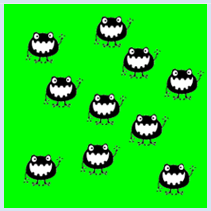It especially makes sense to use OOP when you are using multiple objects of the same class. To experience this close up, a new animal should pop up in your program at each mouse click.

```from gamegrid import *

# ---------------- class Animal ----------------
class Animal():
def __init__(self, imgPath):
self.imagePath = imgPath  # Instance variable
def showMe(self, x, y):  # Method definition
bg.drawImage(self.imagePath, x, y)

def pressCallback(e):
myAnimal = Animal("sprites/animal.gif") # Object creation
myAnimal.showMe(e.getX(), e.getY())  # Method call

makeGameGrid(600, 600, 1, False, mousePressed = pressCallback)
setBgColor(Color.green)
show()
doRun()
bg = getBg()
```
Highlight program code (Ctrl+C to copy, Ctrl+V to paste)

### MEMO

 The properties or attributes of an object are defined as instance variables. They have individual values for each object of the class. Accessing instance variables inside of the class is done by prepending self. From outside the class, you access the attribute by the instance name prefix, e.g. myInstance.attribute. A class has also access to the variables and functions of the main part of the program, for example all methods of the class GameGrid and with bg the backgroundof the game window. The methods can even modify a variable of the main part, if it is declared as global in the method. If the object does not require initialization, the definition of the constructor can also be omitted. Instead of passing the sprite image to the constructor, use the variable imagePath in the following program so that you can forego the constructor. ```from gamegrid import * import random # ---------------- class Animal ---------------- class Animal(): def showMe(self, x, y): bg.drawImage(imagePath, x, y) def pressCallback(e): myAnimal = Animal() myAnimal.showMe(e.getX(), e.getY()) imagePath = "sprites/animal.gif" makeGameGrid(600, 600, 1, False, mousePressed = pressCallback) setBgColor(Color.green) show() doRun() bg = getBg() ```

###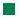Class hierarchies are created through a class derivation or an inheritance, and with it you can add additional properties and behaviors to an existing class. Objects of the derived class are also automatically objects of the parent class (also called base class or super class) and can therefore use all the properties and methods of the parent class as if they were defined in the derived class itself.
 For example, a pet is an animal that also has its own name, which it is should write out using tell(). Hence, you define a class Pet that is derived from Animal. Since you want to specify the name of the animals for each pet individually during its creation, you provide it to the constructor of Pet as an initialization value, which then stores it in an instance variable.```from gamegrid import *
from java.awt import Point

# ---------------- class Animal ----------------
class Animal():
def __init__(self, imgPath):
self.imagePath = imgPath
def showMe(self, x, y):
bg.drawImage(self.imagePath, x, y)

# ---------------- class Pet ----------------
class Pet(Animal):   # Derived from Animal
def __init__(self, imgPath, name):
self.imagePath = imgPath
self.name = name
def tell(self, x, y): # Additional method
bg.drawText(self.name, Point(x, y))

makeGameGrid(600, 600, 1, False)
setBgColor(Color.green)
show()
doRun()
bg = getBg()
bg.setPaintColor(Color.black)

for i in range(5):
myPet = Pet("sprites/pet.gif", "Trixi")
myPet.showMe(50 + 100 * i, 100)
myPet.tell(72 + 100 * i, 145)
```
Highlight program code (Ctrl+C to copy, Ctrl+V to paste)

### MEMO

 As you can see, you can call myPet.showMe() even though showMe() is not defined in the class Pet, because a pet is also an animal. The relationship of Pet and Animal is therefore called an is-a relationship. Since imagePath is set by the Animal constructor, you may replace the line self.imagePath = imgPath in the Pet constructor by Animal.__init__(self, imagePath) to initialize the Animal base class. For derived classes, the base classes are placed in parentheses after the class name. In Python you can also derive a class from several base classes (multiple inheritance).

### CLASS HIERARCHIES, OVERRIDING METHODS

 Methods of the base class can also be changed in a derived class by being redefined (overridden) with the same name and parameter list. If you want to model dogs that also bark with tell(), derive the class Dog from Pet and override the method tell(). You can get a cat to meow by deriving a class Cat from Pet and overriding tell() there as well.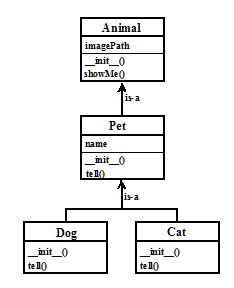The four classes can be visualized in a class diagram. The is-a relationship becomes particularly clear with it [more...In object-oriented software modeling can even automatically from class diagrams a code skeleton are generated. The dominant process is called UML (Unified Modeling Language)]. The classes are displayed as a rectangular box in the class diagram, into which you first write the class name. The instance variables follow separated by a horizontal dividing line and then, led by the constructor, follow the methods of the class. The class hierarchy is easy to follow thanks to a clever arrangement and connecting arrows.
```from gamegrid import *

# ---------------- class Animal ----------------
class Animal():
def __init__(self, imgPath):
self.imagePath = imgPath
def showMe(self, x, y):
bg.drawImage(self.imagePath, x, y)

# ---------------- class Pet ----------------
class Pet(Animal):
def __init__(self, imgPath, name):
Animal.__init__(self, imgPath)
self.name = name
def tell(self, x, y):
bg.drawText(self.name, Point(x, y))

# ---------------- class Dog ----------------
class Dog(Pet):
def __init__(self, imgPath, name):
Pet.__init__(self, imgPath, name)
def tell(self, x, y): # Overriding
bg.setPaintColor(Color.blue)
bg.drawText(self.name + " tells 'Waoh'", Point(x, y))

# ---------------- class Cat ----------------
class Cat(Pet):
def __init__(self, imgPath, name):
Pet.__init__(self, imgPath, name)
def tell(self, x, y): # Overriding
bg.setPaintColor(Color.gray)
bg.drawText(self.name + "  tells 'Meow'", Point(x, y))

makeGameGrid(600, 600, 1, False)
setBgColor(Color.green)
show()
doRun()
bg = getBg()

alex = Dog("sprites/dog.gif", "Alex")
alex.showMe(100, 100)
alex.tell(200, 130)  # Overriden method is called

rex = Dog("sprites/dog.gif", "Rex")
rex.showMe(100, 300)
rex.tell(200, 330)  # Overriden method is called

xara = Cat("sprites/cat.gif", "Xara")
xara.showMe(100, 500)
xara.tell(200, 530)  # Overriden method is called
```
Highlight program code (Ctrl+C to copy, Ctrl+V to paste)

### MEMO

 By overriding methods, you can change the behavior of the base class in the derived classes. When calling methods of the same class or the base class, you have to prepend self. However, self does not have to be provided in the parameter list. Sometimes you might want to use the identical method of the base class in an override method. To invoke it, you have to prefix the class name of the base class and provide self  in the parameter list [more... If you forget this rule, a recursive call would]. This rule also applies to the constructor: if the constructor of the base class is used in the constructor of the derived class, it has to be called by prepending the class name of the base class and passing the parameter self. For example: ```class BaseClass: def __init__(self, a): self.a = a class ChildClass(BaseClass): def __init__(self, a, b): # the initialization of the instance variable 'a' # is delegated to the constructor of the base class BaseClass.__init__(self, a) self.b = b ```

### TYPE-BASED METHOD CALLS: POLYMORPHISM

Polymorphism is a bit more difficult to understand, but it is a particularly important feature of object-oriented programming. It refers to the calling of overridden methods, where the call is automatically adjusted to the class affiliation. With a simple example you can see what this means. You use a list Animals with the previously defined classes in which there are two dogs and a cat

```animals = [Dog(), Dog(), Cat()]
```

A problem occurs when going through the list and calling tell() because there are three different methods of tell()  (one in the class Pet, Dog and Cat).

 ```for animal in animals: animal.tell() ``` The computer can resolve this ambiguity in one of three ways: It can give an error message. It can call tell() of the base class Pet. It can find out what kind of pets you have and then call the appropriate tell(). In a polymorphic programming language such as Python, the last and best option applies.
```from gamegrid import *
from soundsystem import *

# ---------------- class Animal ----------------
class Animal():
def __init__(self, imgPath):
self.imagePath = imgPath
def showMe(self, x, y):
bg.drawImage(self.imagePath, x, y)

# ---------------- class Pet ----------------
class Pet(Animal):
def __init__(self, imgPath, name):
Animal.__init__(self, imgPath)
self.name = name
def tell(self, x, y):
bg.drawText(self.name, Point(x, y))

# ---------------- class Dog ----------------
class Dog(Pet):
def __init__(self, imgPath, name):
Pet.__init__(self, imgPath, name)
def tell(self, x, y): # Overridden
Pet.tell(self, x, y)
openSoundPlayer("wav/dog.wav")
play()

# ---------------- class Cat ----------------
class Cat(Pet):
def __init__(self, imgPath, name):
Pet.__init__(self, imgPath, name)
def tell(self, x, y): # Overridden
Pet.tell(self, x, y)
openSoundPlayer("wav/cat.wav")
play()

makeGameGrid(600, 600, 1, False)
setBgColor(Color.green)
show()
doRun()
bg = getBg()

animals = [Dog("sprites/dog.gif", "Alex"),
Dog("sprites/dog.gif", "Rex"),
Cat("sprites/cat.gif", "Xara")]

y = 100
for animal in animals:
animal.showMe(100, y)
animal.tell(200, y + 30)    # Which tell()????
show()
y = y + 200
delay(1000)
```
Highlight program code (Ctrl+C to copy, Ctrl+V to paste)

### MEMO

 Polymorphism ensures that the class affiliation decides which method is called in overridden methods. Since the affiliation to classes in Python is only determined at runtime anyway, polymorphism is self-evident. The dynamic data binding of Python is called duck test or duck typing, according to the quote attributed to James Whitcomb Riley (1849 – 1916): “When I see a bird that walks like a duck and swims like a duck and quacks like a duck, I call that bird a duck.” There are some cases where an overridden method is defined in the base class, but it should not do anything. This can be achieved either with an immediate return or with the empty statement pass.

### EXERCISES

1.

Define a class TurtleKid derived from the class Turtle that draws a square with shape(). Try to get the following main part to work:

 ```tf = TurtleFrame() # john is a Turtle john = Turtle(tf) # john knows all commands of Turtle john.setColor("green") john.forward(100) john.right(90) john.forward(100) # laura is a TurtleKid, but also a Turtle # laura knows all commands of Turtle laura = TurtleKid(tf) laura.setColor("red") laura.left(45) laura.forward(100) # laura knows a new command too laura.shape() ```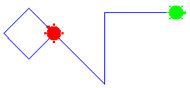2.

Define two derived classes, TurtleBoy and TurtleGirl, from TurtleKid which override  shape() so that a TurtleBoy draws a solid triangle and a TurtleGirl draws a solid circle. The following main part has to work:

 ```tf = TurtleFrame() aGirl = TurtleGirl(tf) aGirl.setColor("red") aGirl.left(45) aGirl.forward(100) aGirl.shape() aBoy = TurtleBoy(tf) aBoy.setColor("green") aBoy.right(45) aBoy.forward(100) aBoy.shape() aKid = TurtleKid(tf) aKid.back(100) aKid.left(45) aKid.shape() ```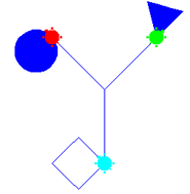3.

Draw the class diagram for exercise 2.

### STATIC VARIABLES AND STATIC METHODS

 Classes can also be used to group related variables or functions, thus making the code easier to read. For example, you can condense the main physical constants in the class Physics. Variables defined in the same class header are called static variables and we call them by prefixing the class name. Unlike with instance variables, it is not necessary to create an instance of the class. ```import math # ---------------- class Physics ---------------- class Physics(): # Avagadro constant [mol-1] N_AVAGADRO = 6.0221419947e23 # Boltzmann constant [J K-1] K_BOLTZMANN = 1.380650324e-23 # Planck constant [J s] H_PLANCK = 6.6260687652e-34; # Speed of light in vacuo [m s-1] C_LIGHT = 2.99792458e8 # Molar gas constant [K-1 mol-1] R_GAS = 8.31447215 # Faraday constant [C mol-1] F_FARADAY = 9.6485341539e4; # Absolute zero [Celsius] T_ABS = -273.15 # Charge on the electron [C] Q_ELECTRON = -1.60217646263e-19 # Electrical permittivity of free space [F m-1] EPSILON_0 = 8.854187817e-12 # Magnetic permeability of free space [ 4p10-7 H m-1 (N A-2)] MU_0 = math.pi*4.0e-7 c = 1 / math.sqrt(Physics.EPSILON_0 * Physics.MU_0) print("Speed of light (calulated): %s m/s" %c) print("Speed of light (table): %s m/s" %Physics.C_LIGHT) ``` Highlight program code (Ctrl+C to copy, Ctrl+V to paste) You can also group a collection of related functions by defining them as static methods in a meaningfully designated class. You can use these methods by directly prepending the class name, without having to create an instance of the class. To make a static method, you have to write the line @staticmethod before the definition. ```# ---------------- class OhmsLaw ---------------- class OhmsLaw(): @staticmethod def U(R, I): return R * I @staticmethod def I(U, R): return U / R @staticmethod def R(U, I): return U / I r = 10 i = 1.5 u = OhmsLaw.U(r, i) print("Voltage = %s V" %u) ``` Highlight program code (Ctrl+C to copy, Ctrl+V to paste)

### MEMO

 Static variables (as opposed to instance variables, also called class variables) belong to the class as a whole and in contrast to instance variables, all objects of the class have the same value. They can be read and changed with prepended class names. A typical use of static variables is an instance counter, which is a variable that counts the number of generated objects of the relevant class. Related functions can be grouped as static methods in a suggestively designated class. The line @staticmethod (called a function decorator) must be prepended when defining the function.